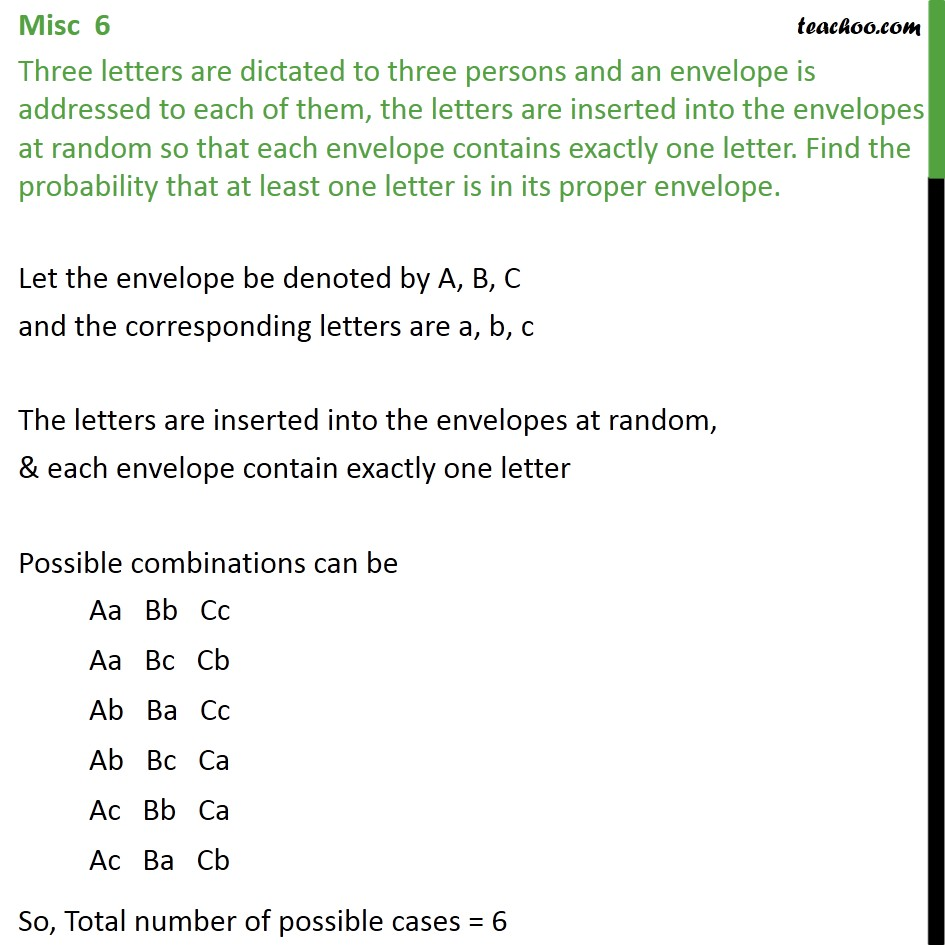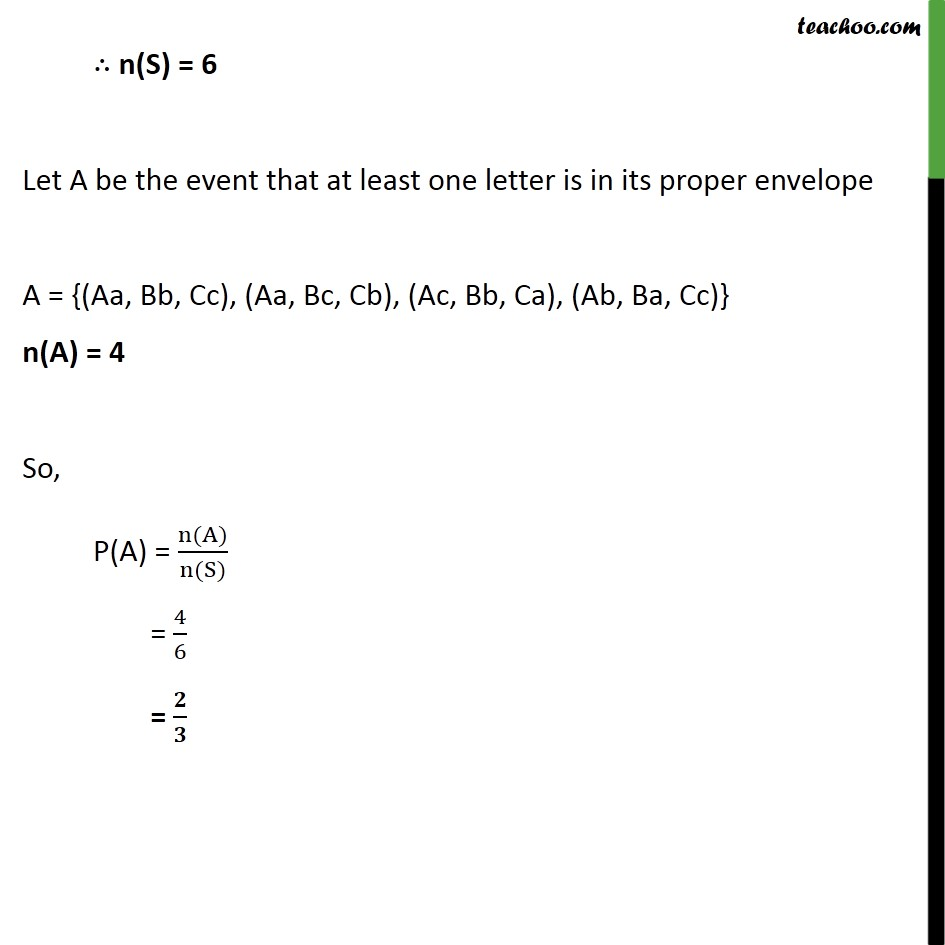Miscellaneous

Chapter 14 Class 11 Probability
Serial order wiseLearn in your speed, with individual attention - Teachoo Maths 1-on-1 Class

### Transcript

Misc 6 Three letters are dictated to three persons and an envelope is addressed to each of them, the letters are inserted into the envelopes at random so that each envelope contains exactly one letter. Find the probability that at least one letter is in its proper envelope. Let the envelope be denoted by A, B, C and the corresponding letters are a, b, c The letters are inserted into the envelopes at random, & each envelope contain exactly one letter Possible combinations can be Aa Bb Cc Aa Bc Cb Ab Ba Cc Ab Bc Ca Ac Bb Ca Ac Ba Cb So, Total number of possible cases = 6 n(S) = 6 Let A be the event that at least one letter is in its proper envelope A = {(Aa, Bb, Cc), (Aa, Bc, Cb), (Ac, Bb, Ca), (Ab, Ba, Cc)} n(A) = 4 So, P(A) = n(A) n(S) = 4 6 =